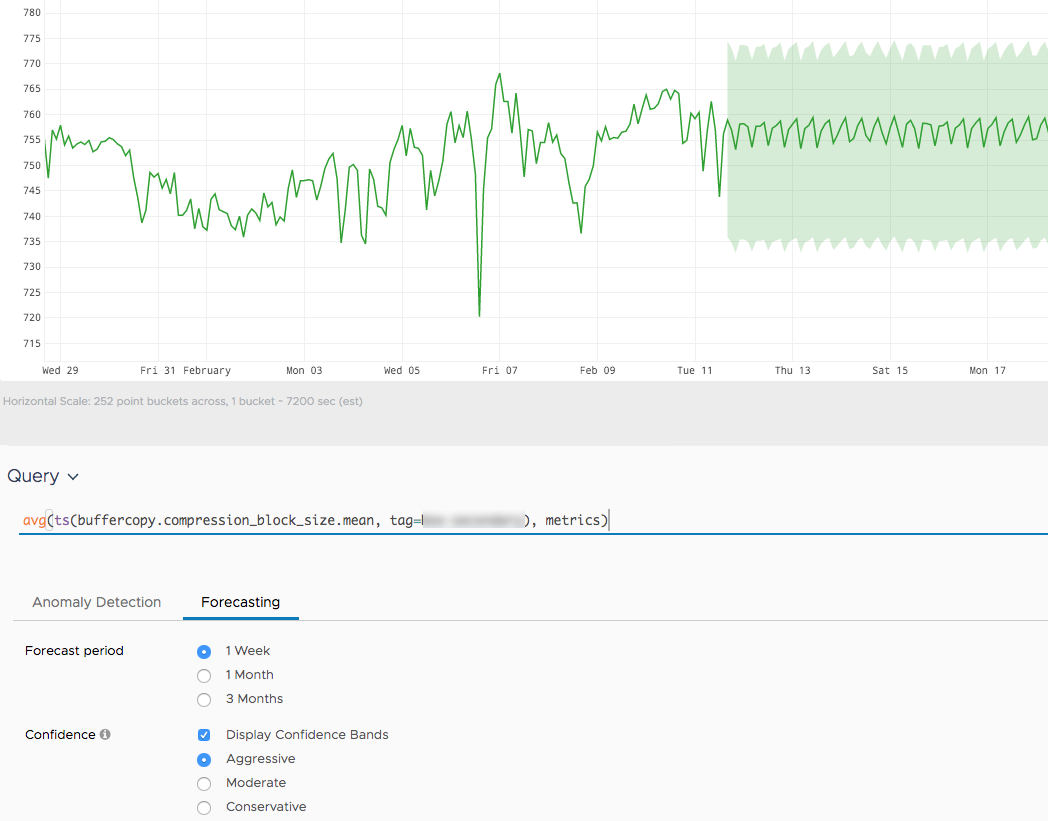Reference to the nnforecast() function

## Summary

``````nnforecast(<forecastPeriod>, [<confidenceFactor>,] <tsExpression>, [with_bounds])
``````

Forecasts future data values for each time series described by the expression. It uses hypothesis testing and neural networks for prediction.

## Parameters

ParameterDescription
forecastPeriod Period of time for the forecast.
confidenceFactor A number from 0.0 to 1.00 (inclusive) that expresses the confidence factor for determining the range of expected values. Default is 0.67. The Note. We consider this parameter only if the with_bounds parameter is also specified.
tsExpression Expression describing the time series to forecast.
with_bounds Enables confidence bounds. Disabled by default.

## Description

The `nnforecast()` function predicts future data values for each time series described by the expression. The function uses hypothesis testing and neural networks for prediction.

In charts, we adjust the time window so that you see the forecast in 1/3 of the window and the past in 2/3 of the window. In the following screenshot, the forecast period is set to 1 week so the total time window is 3 weeks.The historical period has to be at least 2x the forecast period. If the function cannot find enough historical data to produce a forecast for the requested period, then the function returns no result.

## Examples

`nnforecast(7d, ts(my.metric))`

Simple forecasting of 7 days without confidence bounds.

`nnforecast(30d, 0.9, ts(my.metric), with_bounds)`

Forecasting of 30 days with confidence bounds enabled and with a confidence factor of 0.9.